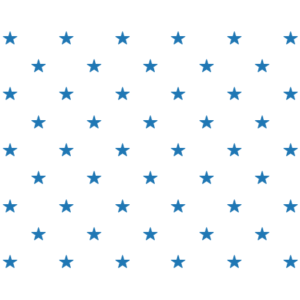# Squaring the pentagon

This week’s Riddler Classic is a number theory problem, which I will paraphrase:

The number 50 can be represented using a set of interleaved dots where the number of columns is one greater than the number of rows; the same way the stars in the US flag are arranged. If we add 1 to this number, we obtain 51, which can be represented using concentric pentagons. Here are diagrams showing both arrangements:What is the next number with the property that it can be represented as interleaved dots but when you add 1 to it it can be represented using concentric pentagons?

Here is my solution:
[Show Solution]

## 2 thoughts on “Squaring the pentagon”

1.Ben Lambert says:

This is a great blog. Thanks for taking the time to provide all of this as a public service.

1.Laurent says:

You’re welcome!